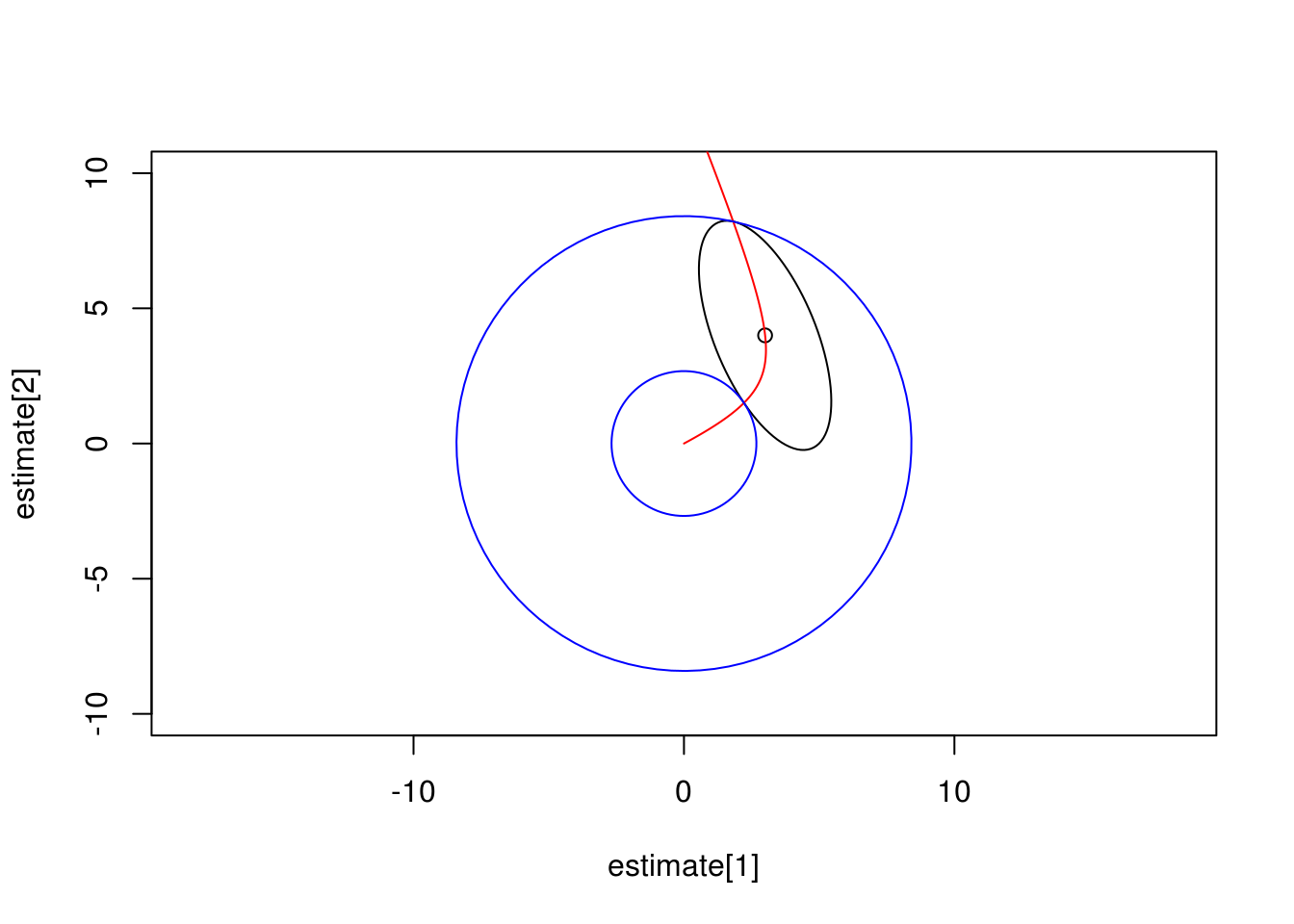# Ball hypothesis

I want to generalize the idea of interval null hypotheses to ANOVA-style tests. Suppose we have estimated a vector $$\hat\theta$$, with error multivariate-normal with covariance matrix $$\Sigma$$. For example, this might be a collection of contrasts on a linear model. Our null hypothesis is that $$\theta$$ lies within a ball of radius $$r$$ centered at zero.

We have the constraint on the null hypothesis $$\theta$$

$\theta^\top \theta \leq r^2$

We’ll seek a worst-case $$\theta$$ within this ball from which to perfrom a chi-square or F test. This will be the point minimizing

$\chi^2 = (\hat\theta - \theta)^\top \Sigma^{-1} (\hat\theta-\theta)$

### Rotate point of view

It will make things easier to rotate our view so that $$\Sigma$$ is a diagonal matrix. Use the eigendecomposition

$\Sigma = QDQ^\top$

and rotate by $$Q^\top$$

\begin{align} \hat\theta &\leftarrow Q^\top \hat\theta \\ \Sigma &\leftarrow D \end{align}

### Constrained optimization

If $$|\theta| \leq r$$, $$\chi^2$$ will be zero. Otherwise we need to locate the optimal $$\theta$$ on the surface of the ball. This can be done using a Lagrange multiplier.

\begin{align} \mathcal{L}(\theta,\lambda) &= (\hat\theta - \theta)^\top \Sigma^{-1} (\hat\theta-\theta) + \lambda (\theta^\top \theta - r^2) \\ &= \hat\theta^\top \Sigma^{-1} \hat\theta -2 \hat\theta^\top \Sigma^{-1} \theta + \theta^\top\Sigma^{-1}\theta + \lambda \theta^\top I \theta - \lambda r^2 \\ &= \hat\theta^\top \Sigma^{-1} \hat\theta -2 \hat\theta^\top \Sigma^{-1} \theta + \theta^\top(\Sigma^{-1}+\lambda I) \theta - \lambda r^2 \end{align}

For a given $$\lambda$$ this is a quadratic form that has a minmum at

$\theta = (\Sigma^{-1}+\lambda I)^{-1} \Sigma^{-1} \hat\theta$

The matrices in this are diagonal, so it can be calculated quickly. I use numerical root finding to choose $$\lambda$$ that places $$\theta$$ on the surface of the ball. It may be more convenient to optimize $$w \in [0,1]$$, $$\lambda=(1-w)/w$$.

$\theta = w(w\Sigma^{-1}+(1-w)I)^{-1}\Sigma^{-1}\hat\theta$

### Test inversion

The above can be used to test a hypothesis for a fixed size ball. It can also be used to calculate the bounds on $$|\theta|$$ for the confidence ellipsoid around $$\hat\theta$$.

### Example in R

#### Confidence interval

library(ellipse)

estimate <- c(3,4)
vcovar <- rbind(
c( 1, -1),
c(-1,  3))

# Plot 95% confidence ellipse
plot(estimate,estimate, xlim=c(-10,10), ylim=c(-10,10), asp=1)
lines(ellipse(vcovar, centre=estimate, level=0.95))

# Rotate point of view
decomp <- eigen(vcovar)
Q <- decomp$vectors rot_estimate <- t(Q) %*% estimate d <- decomp$values

# Functions to calculate theta as a function of w, etc
rot_theta <- function(w) w/(w/d+1-w)/d*rot_estimate
theta <- function(w) Q %*% rot_theta(w)
chisq <- function(w) sum((rot_theta(w)-rot_estimate)^2/d)

# Show the path theta(w) takes
# - First work out where theta shoots off to infinity, and stop a little short of that
w_max <- 1/(1-1/d)
w_max <- min(w_max[w_max>0]) - 1e-3
curve <- sapply(seq(0,w_max,by=1e-3), theta)
lines(curve[1,],curve[2,], col="red")

# Calculate lower and upper bounds on |theta|
critical <- qchisq(0.95, length(estimate))

lower_w <- uniroot(function(w) chisq(w)-critical, interval=c(0,1))$root lower <- radius(lower_w) lines(ellipse(diag(2),t=lower), col="blue") upper_w <- uniroot(function(w) chisq(w)-critical, interval=c(1,w_max))$root
lines(ellipse(diag(2),t=upper), col="blue")Black ellipse is confidence ellipse. Blue circles are lower and upper confidence limits on $$|\theta|$$. Red line is the path taken by $$\theta(w)$$.

cat(sprintf("|estimate|=%.2f, 95%% CI [%.2f,%.2f]\n", sqrt(sum(estimate^2)), lower, upper))
## |estimate|=5.00, 95% CI [2.68,8.41]

#### p-value

# p-value for specific radius

cat(sprintf("Test of hypothesis that |theta|<=%.2f: p=%.4f\n", test_radius, p))
## Test of hypothesis that |theta|<=2.00: p=0.0047